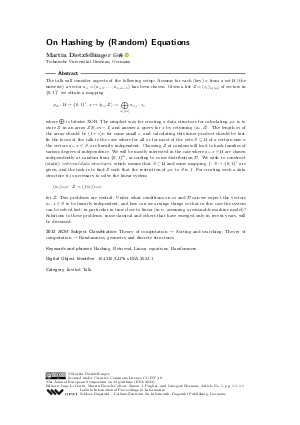Document# On Hashing by (Random) Equations (Invited Talk)

### Author Martin Dietzfelbinger## File

LIPIcs.ESA.2023.1.pdf
• Filesize: 392 kB
• 1 pages

## Cite As

Martin Dietzfelbinger. On Hashing by (Random) Equations (Invited Talk). In 31st Annual European Symposium on Algorithms (ESA 2023). Leibniz International Proceedings in Informatics (LIPIcs), Volume 274, p. 1:1, Schloss Dagstuhl - Leibniz-Zentrum für Informatik (2023)
https://doi.org/10.4230/LIPIcs.ESA.2023.1

## Abstract

The talk will consider aspects of the following setup: Assume for each (key) x from a set 𝒰 (the universe) a vector a_x = (a_{x,0},… ,a_{x,{m-1}}) has been chosen. Given a list Z = (z_i)_{i ∈ [m]} of vectors in {0,1}^r we obtain a mapping φ_Z: 𝒰 → {0,1}^r, x ↦ ⟨a_x,Z⟩ := ⨁_{i ∈ [m]} a_{x,i} ⋅ z_i, where ⨁ is bitwise XOR. The simplest way for creating a data structure for calculating φ_Z is to store Z in an array Z[0..m-1] and answer a query for x by returning ⟨ a_x,Z⟩. The length m of the array should be (1+ε)n for some small ε, and calculating this inner product should be fast. In the focus of the talk is the case where for all or for most of the sets S ⊆ 𝒰 of a certain size n the vectors a_x, x ∈ S, are linearly independent. Choosing Z at random will lead to hash families of various degrees of independence. We will be mostly interested in the case where a_x, x ∈ 𝒰 are chosen independently at random from {0,1}^m, according to some distribution 𝒟. We wish to construct (static) retrieval data structures, which means that S ⊂ 𝒰 and some mapping f: S → {0,1}^r are given, and the task is to find Z such that the restriction of φ_Z to S is f. For creating such a data structure it is necessary to solve the linear system (a_x)_{x ∈ S} ⋅ Z = (f(x))_{x ∈ S} for Z. Two problems are central: Under what conditions on m and 𝒟 can we expect the vectors a_x, x ∈ S to be linearly independent, and how can we arrange things so that in this case the system can be solved fast, in particular in time close to linear (in n, assuming a reasonable machine model)? Solutions to these problems, some classical and others that have emerged only in recent years, will be discussed.

## Subject Classification

##### ACM Subject Classification
• Theory of computation → Sorting and searching
• Theory of computation → Randomness, geometry and discrete structures
##### Keywords
• Hashing
• Retrieval
• Linear equations
• Randomness

## Metrics

• Access Statistics
• Total Accesses (updated on a weekly basis)
0